# Algebra 1 : How to find mode

## Example Questions

### Example Question #51 : How To Find Mode

What is the mode of the following set of numbers?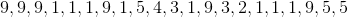Explanation:

We define mode as the number that appears most often in a set of numbers. Examining the set above, we see thatappears the most times and is therefore the mode of the set.

### Example Question #52 : How To Find Mode

What is the mode of the following set of numbers?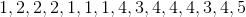Explanation:

We define mode as the number that appears most often in a set of numbers. Examining the set above, we see thatappears the most times and is therefore the mode of the set.

### Example Question #53 : How To Find Mode

What is the mode of the following set of numbers?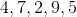No ModeNo Mode

Explanation:

We define mode as the number that appears most often in a set of numbers. Examining the set above, we see that each number appears only once; therefore, the set has no mode

### Example Question #51 : How To Find Mode

What is the mode of the following set of numbers?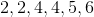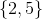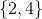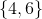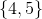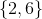Explanation:

We define mode as the number that appears most often in a set of numbers. Examining the set above, we see that bothandappear two times, which is the most of any number in the set. Therefore, the set is bimodal and has two modes,.

### Example Question #52 : How To Find Mode

What is the mode of the following set of numbers?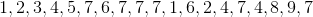Explanation:

We define mode as the number that appears most often in a set of numbers.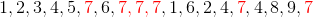Examining the set above, we see thatappears six times which is the most in the set and is therefore the mode of the set.

### Example Question #52 : How To Find Mode

What is the mode of the following set of numbers?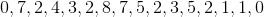Explanation:

We define mode as the number that appears most often in a set of numbers.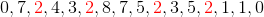Examining the set above, we see thatappears four times which is the most in the set and is therefore the mode of the set. The next highest occurance of any number in this set is two which zero, one, three, five, and seven are all seen twice in the set.

### Example Question #54 : How To Find Mode

What is the mode of the following set of numbers?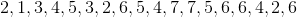Explanation:

We define mode as the number that appears most often in a set of numbers.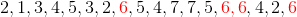Examining the set above, we see thatappears four times which is the most in this set and is therefore the mode of the set.

### Example Question #55 : How To Find Mode

What is the mode of the following set of numbers?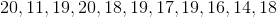Explanation:

We define mode as the number that appears most often in a set of numbers.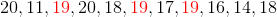Examining the set above, we see thatappears the most times and is therefore the mode of the set.

### Example Question #53 : How To Find Mode

What is the mode of the following set of numbers?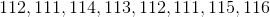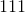and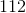None of the above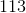andExplanation:

We define mode as the number that appears most often in a set of numbers.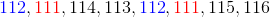Examining the set above, we see thatandappear the most times and are therefore the modes of this (bimodal) set.

### Example Question #60 : How To Find Mode

What is the mode of the following set of numbers?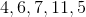No ModeNo Mode

Explanation:

We define mode as the number that appears most often in a set of numbers.Examining the set above, we see that no number appears more often than any other number - therefore, there is no mode in the set.

### All Algebra 1 Resources